kira-s

# Polytopes for Experts

Version s01
Meta
Known topics
Symmetry spaces
Geometric spaces
Definitions
in spaces
Concept
Algorithms
Examples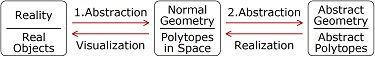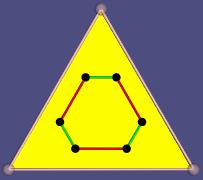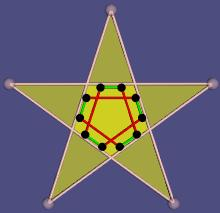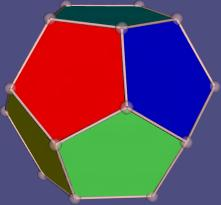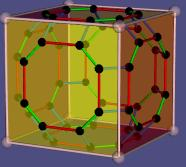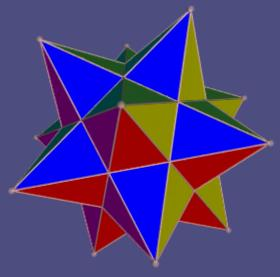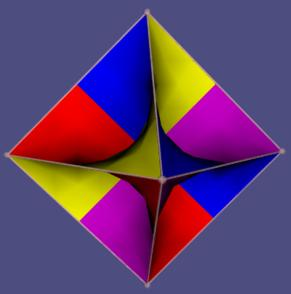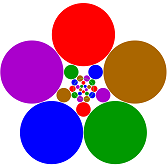• Please click to the red question mark to get help for the navigation.
• Click to words of the graphic for explanation to this part of the graphic. (Does not work with all browsers).
• Click to the small images for larger versions and annotations.

# Abstract

This article is based on abstract polytopes as described in the book "Abstract Regular Polytopes" by Peter McMullen and Egon Schulte. But an alternative, more flexible definition of realizations is used here. The usual realization of an abstract cube {4,3} in space only uses 8 points in the space. The new one can do the same but also the solid cube, the hollow cube, a box open at one side, 8 circles in the plane and other versions. These all can be distinguished in an abstract way. Realizations of infinite 4- and 5-polytopes in the plane and in the 3-space are used for visualizations. Their beauty may get the interest of persons who never would look at a mathematical paper.
This article primarily is written for experts in the theory opf polytopes. But other people are invited to read it too, some help texts are given.

#### What you should know

The term (geometrical) polytope is a generalization of polygon (triangle, rectangle, ...) and poyhedron (three dimensional solid figures with plane borders) to objects in mathematical spaces of other dimensions and types. Polytopes have a dimension n and border elements (called faces) of rank 0 to n-1.

The faces build a partially ordered set (poset). When we look at the posets and ignore the space, we get abstract polytopes. And we get further abstract polytopes as posets with similar properties. Often they can be transformed to mathematical spaces to get new geometrical polytopes. This transformation is called realization.

The usual realization in a space only gives points and the information, how they are combined in the other faces. That may satisfy many mathematicians but not me. Hence I define another, more flexible type of realization. It can give not only the eight vertices of a cube but also the solid cube, the hollow cube and other versions.

I use my realization in mathematical spaces normaly not used for polytopes. Such realizations of up to five dimensional abstract polytopes can be visualized. The beauty of the visualization may get the interest of especially young persons who never would look at a mathematical paper.

# The author

Kira Stöwe in Cologne, Germany, has studied mathematics and physics. She published in theoretical physics and some other fields. Now she researches in mathematical fields for her pleasure, mostly without contact to literature or other peoples. Only seldom she looks what other persons have found and adepts their terms. Results that she does not find elsewhere may be new or overseen. So she describes her results here and looks for a partner for a scientific publication. In addition she wants to communicate the whole theory in a simple way to children and looks for partners.

# The Author

Kira Stöwe ...
1. is a woman in Cologne, Germany
2. has studied mathematics and physics
3. published mainly in theoretical physics
4. now researches in mathematical fields for her pleasure,
mostly without contact to literature or other peoples
5. starting from the platonic solids found many types of polytopes
which, as she later noticed, were found by others too
6. but also dicovered some results that she did not found elsewhere
7. describes the results here and looks for a partner for a scientific publication
8. wants to communicate the whole theory in a simple way to children and looks for partners

## Searching polytopes

Kira Stöwe ...
1. on leave in 1988 asked herself:
"Euclid showed that there are exactly 5 regular convex polyhedra, the platonic solids.
But which objects are there in higher dimensions?"
2. in a week found the answer (6,3,3,..), but wanted more regular objects
3. had years of searching nearly without input from literature or other persons,
4. recognized infinite tessalations of spaces as such objects:
in euklidean spaces {∞}, {4,4}, {3,6}, {6,3}, {4,3,..,4}
in elliptic spaces by identifying opposite elements of the latter ones
in hyperbolic spaces (first seen in graphics by M.C.Escher)
at the end also in extended hyperbolic spaces and in conformal spaces
5. found 9 finite regular polyhedra with skew faces,
but did not recognize Petrie's correspondency to the 9 platonic and Kepler-Poinsot polyhedra
6. being familiar with graph theory made a theory of face graphs and flag graphs
which is equivalent to the definition of abstract polytopes later read in 
7. defined regularity equivalent to the usual one and realization not equivalent to the usual one
8. discovered extensions to hyperbolic spaces and read of conformal spaces
9. found polytopes in extended hyperbolic spaces (hyperideal polytopes)
and their projections in conformal spaces
giving (n-2)-dimensional realizations of n-dimensional abstract polytopes
10. made colored images of these realizations and showed them at kira-s.de and at exhibitions

Kira Stöwe has studied mathematics and physics. She published in theoretical physics and some other fields. Now she researches in mathematical fields for her pleasure, mostly without contact to literature or other peoples. On leave in 1988 she asked herself: "Euclid described the 5 platonic solids and showed that there are no more such objects. But which objects are there in higher dimensions?" In short she had the answer: 6 in 4 dimensions and 3 for every higher dimension. Her interest for regular geo metrical objects grew. She found them in lower dimensions (-1 to 2), in tessalations of the plane {4,4}, {3,6}, {6,3}, of higher Eucliden spaces {4,3,..,4} and - by graphics of M.C.Escher - of the hyperbolic plane.

# Meta - Über diese Arbeit

Entwurf Bildchenleiste: Bildchen
Dreieck
Bild Dreieck rechts>
Erläuterung regelmäßig (vorläufig)
Einführung {3}
Unterbildchen {4},{5},{6},{7}
Pentagramm {5/2}
rechts {5/2} groß
Erläüterung
Unterbildchen {7/2},{7/3},{8/3},{10/3}
Dreieck mit Facegraph
Dreieck mit Chamber-Flaggraph
Hasse-Diagramm
Würfel
auch mit Graphen
Digon {2}
Kreisform, ∞-Form, [3} auf Kreis, {2,6}, {6,2}
Würfel, statt Quadrate je 2 sich selbst schneidene {4}
Ein Flächenpaar, {3,3} mit Kerben
{z4}
{cz4,3}
{3,3,3}
{4,3} und {3,4} in Kreisen
Nachzugucken
Polare bei http://de.wikipedia.org/wiki/Dualit%C3%A4t_%28Mathematik%29

# Known topics

The reader should be aquainted with the following topics:

Mathematical logic, quantors
Set theory
Groups
• Generating subset of a group
• Group action
Linear algebra
• Definition
• Matrices
• Eigenvalues aund -vectors of symmetric matrices
Partial orders
Projections and reflections
• Reflections at circles of the plane and of the sphere
• Transformation of a sphere to a plane:
orthogonal, mid point centered, pole centered, mercator
Geometric spaces
• Euclidean spaces
• Elliptic spaces
• Hyperbolic spaces
• Projective geometry
• Conformal geometry
Convex polytopes
Abstract polytopes
• Definition
• Regularity
• Coxeter Groups
• Representation of universal regular polytopes
• Realization

# Topics

Another definition of realization of an abstract polytope
• The usual definition of the realization of an abstract polytope P into an euclidean space E is given by a function β0:F0→E of the vertices into E and recursively for i=1..n and f∊Fi: βi(f)={βi-1(g) | g∊Fi-1∧g≤f}
We call this definition ∊realization and retrict it to faithful realizations, that is all fi are injective.
• The new Definition uses ⊂ instead of ∊. In the following the word realization means the new ⊂realization.
• To each ∊realization of a lattice polytope corresponds a definite ⊂realization. But there are further ⊂realizations.
• To each realization of a polytope corresponds a realization of the dual.
• A polytope of range n may have realizations of dimension n-2.
• The different realizations of an abstract polytope P can be characterized in an abstract way.
Realizations in unusual spaces
• Extended hyperbolic spaces
• Conformal spaces
Visualzation of realizations
• Calculation of a representation
• Projections
• Aesthetics

# Symmetry spaces

All spaces that usually are used for polytopes have a set of points and symmetries. So I abstract:
Definition
• A symmetry space is a pair (M,S) of a set M and of+ a set S of bijections M→M that form a group.
• The elements are called points and (space-)symmetries.
Fixpoints, Orbits, Corunners, subspaces
fixpoints (x is a fixpoint :⇔ ∀s∊S s(x)=x), orbit (orbit(x,s), Orbit(M',S')) corunners (x,y are corunners :⇔ ∀s∊S (s(x)=x ⇔ s(y)=y), bunch (pulk: a class of corunners in an S-orbit), S-orbitclass (orbit in the same class, if they have corunners), poset of subspaces

• Symmetry spaces are similar to group actions.
• In this article symmetry spaces are used for realizations of abstract polytopes.
• Trivial spaces are (M,S) with S containig only the identity or all bijections or all bijections that change only a finite set.
• For up to 2 elements in M the (M,S) are all trivial:
1. (∅,{∅}}
2. {{x},{{(x,x)}}}
3. {{x,y},{{(x,x),(y,y)}}} and {{x,y},{{(x,x),(y,y)},{(x,y),(y,x)}}}
• Many usual spaces are symmetry spaces: linear, euklidean, spherical, elliptic, hyperbolic, extended hyperbolic, conformal.
• A topological symmetry space (M,T,S) has a toplogy (M,T) and set S of homeomorphisms that forms a group.

## Isomorphims and homomorphisms

Definitions:
• A isomorphism/homomorphism from space (M,S) to space (M',S') is a bijective/surjective function f:M→M' and a bijective/surjective function g:S→S' with
s∊S g(s)={(f(a),f(b))|(a,b)∊s}
• An automorphism in (M,S) is an isomorphism (M,S)→(M,S). It is strong, if g is the identity.
• The space (M,S) is called homomorphic / isomorphic to the space (M',S') if there is a homomorphism / isomorphism.
Implications:
• (M,S) is isomorphic to (M',S') ⇔ (M',S') is isomorphic to (M,S)
So we may say: (M,S) and (M',S') are isomorph.
• If (M,S) is homomorphic to (M',S') and vice versa, then they are isomorphic.
(The author could prove this only with the axiom of choice.)

## Symmetries of parts (part-symmetries)

Definition
• For a symmetry space (M,S) and a set M'⊆M (the part) the set of symmetries of M' is
S'=sym(M,S,M'):={s∩(M'×M')|s∊S ∧ s^(M')=M' ∧ s-1^(M')=M'}
(For s^ see Notations.)
Implications
• (M',S') is a symmetry space
• If M'=M then S'=S.

## Fixpoints

A fixpoint x of (M,S) fullfills ∀s∊Ss(x)=x.

## Orbits

Definition
• For x∊M and s∊S the s-orbit of x (orbit(x,s)) is the smallest set o with x∊o and s^(o)=o and s-1^(o)=o.
• For x∊M the S-orbit of x (orbit(x,S)) is the smallest set o with x∊o ∧ ∀ss∊S (s^(o)=o ∧ s-1^(o)=o).

## Corunners

Definition
• x,y∊M (x and y are corunners) :⇔ (∀s∊S (s(x)=x ⇔ s(y)=y))
• The set of corunners of x in the same S-orbit is called bunch(x).

## Subspaces

Definition
• A subspace or symmetry subspace (sss) of (M,S) is a pair (M',S') with a set M'⊆M, its symmetries S'=sym(M,S,M') and the conditions
1. x∊M', s∊S (s(x)∊M' ⇒ s∩(M'×M')∊S')
2. x∊Ms∊S x∊s^(M')
• The subspace (M',S') of (M,S) is proper if it is not isomorphic to (M,S).

• The definition is intended to give similar subspaces as the usual special definition, but there are discrepancies. So we sometimes destinct "symmetry subspaces" und "usual subspaces". Maybe a better definition of symmetric subspace can be found.
• Each symmetry space (M,S) is a subspace of itself.
• The empty symmetry space (∅,{∅}} is no subspace of other symmetry spaces.

Linear spaces
y
Affine spaces
y

# Geometric spaces

The examples have small dimensiosn, but can be extended to higher dimensions.
We use the following projections between a unit sphere and a plane:
1. Orthogonal to a plane through the equator or tangent to a pole
2. Central from the north pole to a plane through the equator or tangent to he south pole. The plane is closed by an infinite point.
3. Central from an equator point to a plane through the axis or tangent to the opposite. The plane is closed by an infinite point.
4. Central from the sphere center to a plane tangent to the south pole. The plane is closed by an infinite circle where opposite points are identified.

The following spaces are all symmetry spaces and are used for realizations of polytopes.
Sphere, elliptic plane, conformal plane, euklidean plane,
hyperbolic plane, extended hyperbolic plane, extended hyperbolic 3-space

# Euklidean plane

The space may be realized as an affine space of dimension 2.
The projection 2 transformes the circles of the plane to circles of the sphere and the circles of the plane to circles of the sphere that cross the north pole. The reflection at lines in the sphere are transformed to reflections at these spheres.

# Literature

  Peter McMullen, Egon Schulte: Abstract regular polytopes. Cambridge university press (2002)  Branko Grünbaum: Regular polyhedra - old and new. Aequationes Math. 16(1977), 1-20  Xiliang Bao, Francis Bonahon: Hyperideal polyhedra in hyperbolic 3-space. Bull. Soc. math. France 130 (3), 2002, p. 457-491  H.S.M.Coxeter: The beauty of geometry. Dover publications inc, Minesota, New York, 1968  Article 10 of : Regular honeycoms in hyperbolic space. Reprint of: Proceedings of the international congress of mathematiciants, Amsterdam, 1954 (Vol. III)  Yunhi Cho and Hyuk Kim: The analytic continuation of hyperbolic space. http://arxiv.org/abs/math/0612372v1, 2006  Conformal geometry. G.V. Bushmanova (originator), Encyclopedia of Mathematics. URL: http://www.encyclopediaofmath.org/index.php?title=Conformal_geometry&oldid=16231